# The Gyroelongated Triangular Bicupola

The gyroelongated triangular bicupola is the 44th Johnson solid (J44). It has 18 vertices, 42 edges, and 26 faces (20 equilateral triangles and 6 squares).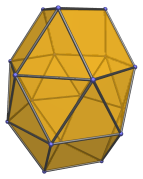The gyroelongated triangular bicupola can be constructed by attaching two triangular bicupolae to a hexagonal antiprism, or equivalently, adding a second triangular cupola to a gyroelongated triangular cupola (J22).

J44 is one of the few Johnson solids that are chiral; it is distinct from its own mirror image: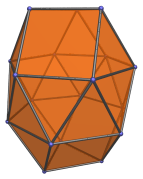## Projections

Here are some views of the gyroelongated triangular bicupola from various angles:

Projection Description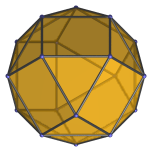Top view.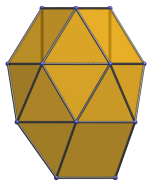Front view.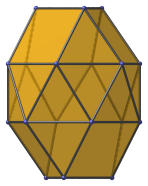15° side view.

## Coordinates

The Cartesian coordinates of the gyroelongated triangular bicupola with edge length 2 are:

• (±1, −1/√3, √(8/3) + √(√3−1))
• (0, 2/√3, √(8/3) + √(√3−1))
• (±1, ±√3, √(√3−1))
• (±2, 0, √(√3−1))
• (±√3, ±1, −√(√3−1))
• (0, ±2, −√(√3−1))
• (−1/√3, ±1, −(√(8/3) + √(√3−1)))
• (2/√3, 0, −(√(8/3) + √(√3−1)))

Last updated 28 May 2018.